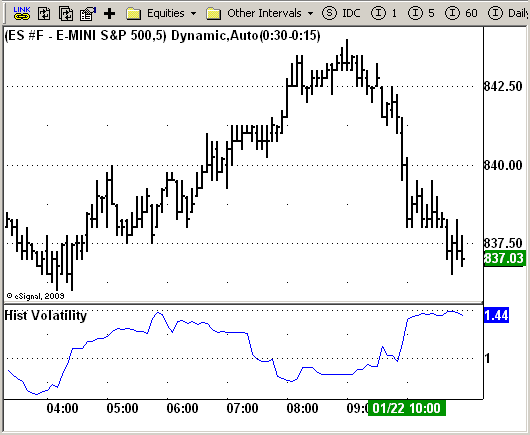# Historical Volatility

ICE Data Services -

HistVolatility.efs   EFSLibrary - Discussion Board

File Name: HistVolatility.efs

Description:
Historical Volatility

Formula Parameters:
LookBack : 20
Annual : 252

Notes:
Markets oscillate from periods of low volatility to high volatility
and back. The author`s research indicates that after periods of
extremely low volatility, volatility tends to increase and price
may move sharply. This increase in volatility tends to correlate
with the beginning of short- to intermediate-term moves in price.
They have found that we can identify which markets are about to make
such a move by measuring the historical volatility and the application
of pattern recognition.
The indicator is calculating as the standard deviation of day-to-day
logarithmic closing price changes expressed as an annualized percentage.

HistVolatility.efsEFS Code:

```//*********************************Provided By:
eSignal (Copyright c eSignal), a division of Interactive Data
Formula Script (EFS) is for educational purposes only and may be
modified and saved under a new file name.  eSignal is not responsible
for the functionality once modified.  eSignal reserves the right
to modify and overwrite this EFS file with each new release.Description:
Hist Volatility
Version:      1.0  01/19/2009
Formula Parameters:
Default:
LookBack 20
Annual   252
Notes:
Markets oscillate from periods of low volatility to high volatility
and back. The author`s research indicates that after periods of
extremely low volatility, volatility tends to increase and price
may move sharply. This increase in volatility tends to correlate
with the beginning of short- to intermediate-term moves in price.
They have found that we can identify which markets are about to make
such a move by measuring the historical volatility and the application
of pattern recognition.
The indicator is calculating as the standard deviation of day-to-day
logarithmic closing price changes expressed as an annualized percentage.
**********************************//
var fpArray = new Array();
var bInit = false;
function preMain() {
setPriceStudy(false);
setStudyTitle("Hist Volatility");
setCursorLabelName("HisVol");
var x = 0;
fpArray[x] = new FunctionParameter("LookBack", FunctionParameter.NUMBER);
with (fpArray[x++]) {
setLowerLimit(1);
setDefault(20);
}
fpArray[x] = new FunctionParameter("Annual", FunctionParameter.NUMBER);
with (fpArray[x++]) {
setLowerLimit(1);
setDefault(252);
}
}
var xPrice1 = null;
var xPrice1Avg = null;
var xStdDev = null;
function main(LookBack, Annual) {
var nState = getBarState();
if (nState == BARSTATE_ALLBARS) {
if (LookBack == null) LookBack = 20;
if (Annual == null) Annual = 252;
}
if (bInit == false) {
xPrice1 = efsInternal("Calc_Price1");
xPrice1Avg = sma(LookBack, xPrice1);
xStdDev = efsInternal("Calc_StdDev", LookBack, xPrice1Avg, xPrice1);
bInit = true;
}
if (getCurrentBarCount() < LookBack + 1) return;
var nAvg = xPrice1Avg.getValue(0);
var HVol = xStdDev.getValue(0) * Math.sqrt(Annual);
return HVol;
}
function Calc_Price1() {
var nRes = 0;
if (close(-1) == null) return;
nRes = Math.log(close(0) / close(-1));
if (nRes == null) nRes = 1;
return nRes;
}
function Calc_StdDev(LookBack, xPrice1Avg, xPrice1) {
var nRes = 0;
if (xPrice1Avg.getValue(0) == null) return;
for (var i = 0; i < LookBack; i++) {
nRes +=
(xPrice1.getValue(-i) - xPrice1Avg.getValue(0)) *
(xPrice1.getValue(-i) - xPrice1Avg.getValue(0));
}
nRes = Math.sqrt(nRes / LookBack);
if (nRes == null) nRes = 1;
return nRes;
}
```